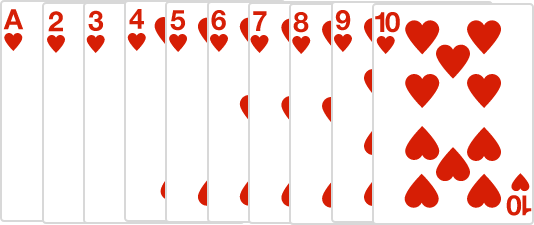# Odd Even Probabilities

Probability Level 2If I randomly pick a card from the ten above (ace = 1), then the probability of it having an odd number or an even number is equal.

If I instead randomly pick two distinct cards from the ten above, is the probability of the two numbers having an odd sum or an even sum still equal?

×

Problem Loading...

Note Loading...

Set Loading...